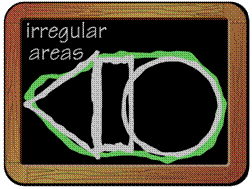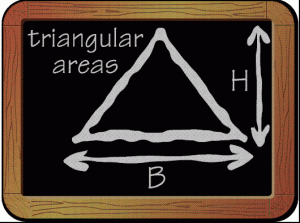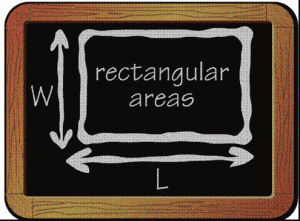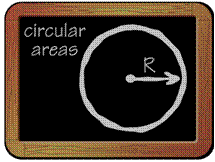# Calculating the Correct Amount

## WHY ARE CALCULATIONS IMPORTANT?

Using just the right amount of pesticide and fertilizer minimizes the potential for personal, property, or environmental damage and maximizes consumers’ return on the dollar. This page will assist you in selecting products and calculating application rates for pesticides and lawn and garden fertilizers commonly used around the home. (adapted from Purdue Pesticide Programs pub. 39)

## HOW DO I CALCULATE THE AREA TO BE TREATED?

Typically, use rates for lawn care products are stated per 1000 square feet or per acre. Rates for flowers, shrubs, and vegetables often are given per 100 square feet. To determine how much pesticide or fertilizer is needed to do a job, first calculate the size of the area to be treatedChances are that you don’t have a “geometrically perfect” area to treat. Irregularly shaped flower beds, etc., usually can be divided into smaller, geometric areas (square, rectangle, circle, triangle) to facilitate total area calculation. Simply calculate the area for each individual shape and add them together.

Conversely, it may be necessary to compute the total square footage of structures within the area which will not be treated—buildings, patios, sidewalks, ponds, etc.—and subtract it from the overall area.

### Calculating Triangular AreasThe equation for calculating the area of a triangle is as follows:

Area = (Base x Height) ¸ 2

If the base of a triangular area is 200 ft and the height is 40 ft, the equation would be

Area = (200 ft x 40 ft) ¸ 2 = 4000 ft2

### Calculating Rectangular AreasThe area of a square or rectangle is determined by multiplying the length times the width:

Area = L x W

A rectangular area measuring 80 ft by 60 ft equals 4800 square feet:

Area = 80 ft x 60 ft = 4800 ft2

### Calculating Circular AreasThe area of a circle is determined by using the equation pr2, or 3.14 x the squared radius of the circle:

Area = 3.14 x r2

A circular flower bed measuring 40 ft in diameter would have a radius of 20 ft, so:

Area = 3.14 x 202

= 3.14 x (20 ft x 20 ft)

= 1,256 ft2

## HOW MUCH DO I NEED TO MIX?

Read the product label for specific instructions on the amount to mix. Some sample calculations follow

### Example A

A product label says to use 3 ounces per 1000 square feet. How much product will you need for a 4800-square-foot area?

4800 ft2 / 1000 ft2 = 4.8 (units of 1000 ft2)

4.8 x 3 oz = 14.4 oz of product

### Example B

A product label prescribes 2 pounds per acre. How much is needed for a 4800-square-foot area? Remember that one acre equals 43,560 square feet.

4800 ft2 / 43,560 ft2 per acre = 0.1 acre

0.1 acre x 2 lb = 0.2 lb of product

0.2 lb x 16 oz = 3.2 oz

### Example C

You need to apply a grub control insecticide over 4800 square feet. The label instructions say to apply 1 gallon of water and 4 teaspoons of insecticide for each 200 square feet. How much water and how much insecticide is needed?

Water needed:

4800 ft2 / 200 ft2 per gal = 24 gal

Insecticide needed:

24 gal x 4 tsp = 96 tsp insecticide per 24 gal water

48 teaspoons equal 1 cup, so convert the 96 tsp to cups, as follows:

96 tsp / 48 tsp = 2 C insecticide per 24 gal of water

### Example D

You need to spread a crabgrass herbicide over a 2400-square-foot area, and you purchase a 25-pound bag of the herbicide. The label states that the contents of the bag will cover 5,000 square feet. How much of the bag will be needed?

2400 ft2 / 5000 ft2 per bag = 0.48 bag

0.48 bag x 25 lb per bag = 12 lb

For more on area and volume calculations

## APPLICATION EQUIPMENT CALIBRATION

Equipment calibration involves the same three steps, regardless of the equipment or pesticide used. Step one; select the proper pesticide and application equipment; step two, determine the size of the treatment area; and step three, determine the correct amount needed.

However, for different pieces of equipment, the process is a little different for each piece.

From the table of links below; click on the equipment you need to calibrate. We’ll show what you need to do.

For additional information on determining the correct amount of pesticide to use:

Pesticide Safety and Calibration Math for the Homeowner (PPP-39). Mar. 2003. Fred Whitford, Andrew Martin, and Roy Ballard. Purdue University Cooperative Extension Service. Pesticide Safety and Calibration Math for the Homeowner by Fred Whitford and Andrew Martin. This online book provided by the Purdue Pesticide Programs has information on How to Choose the Right Pesticide, Why calculations are important, how to calculate the area to be treated and other various topics of interest in applying pesticides.

Home Lawn Basics. Mar. 2009. Brad S. Fresenburg. University of Missouri Extension. It contains quick facts and steps for pesticide sprayers.

Compiled by Wayne Buhler, PhD.